# Find the compound of the formula CroH16, composed of two six-membered rings, that has only three...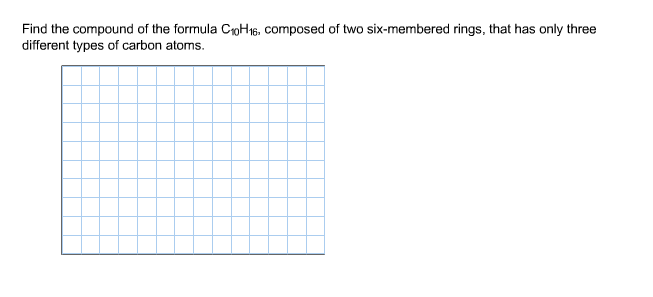Find the compound of the formula CroH16, composed of two six-membered rings, that has only three different types of carbon atoms.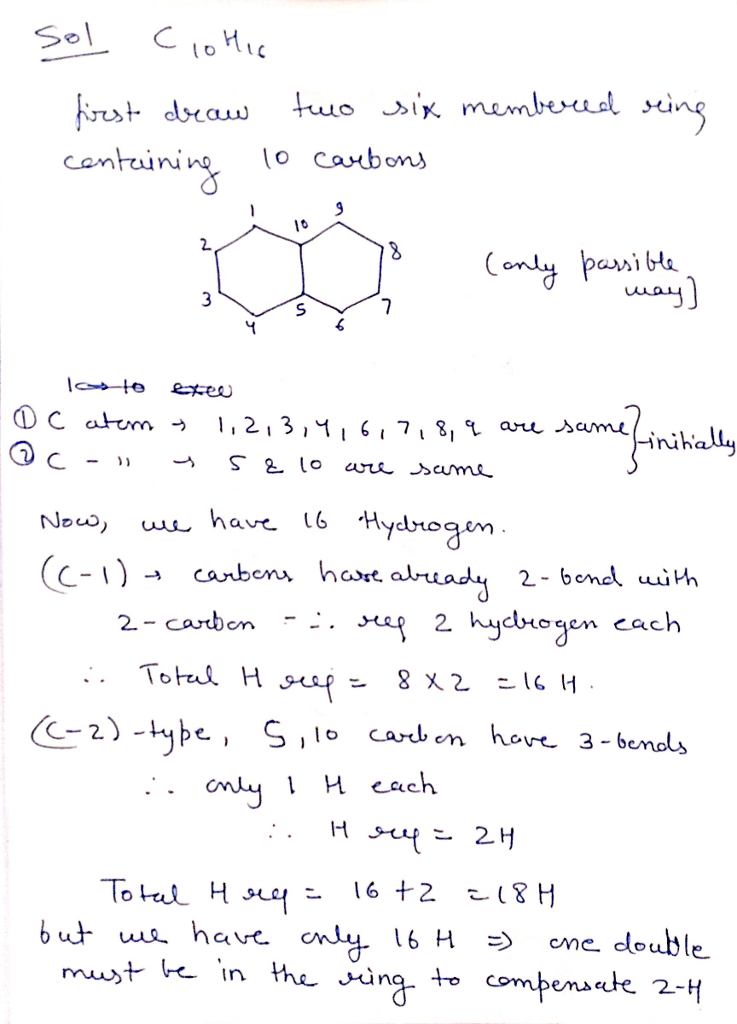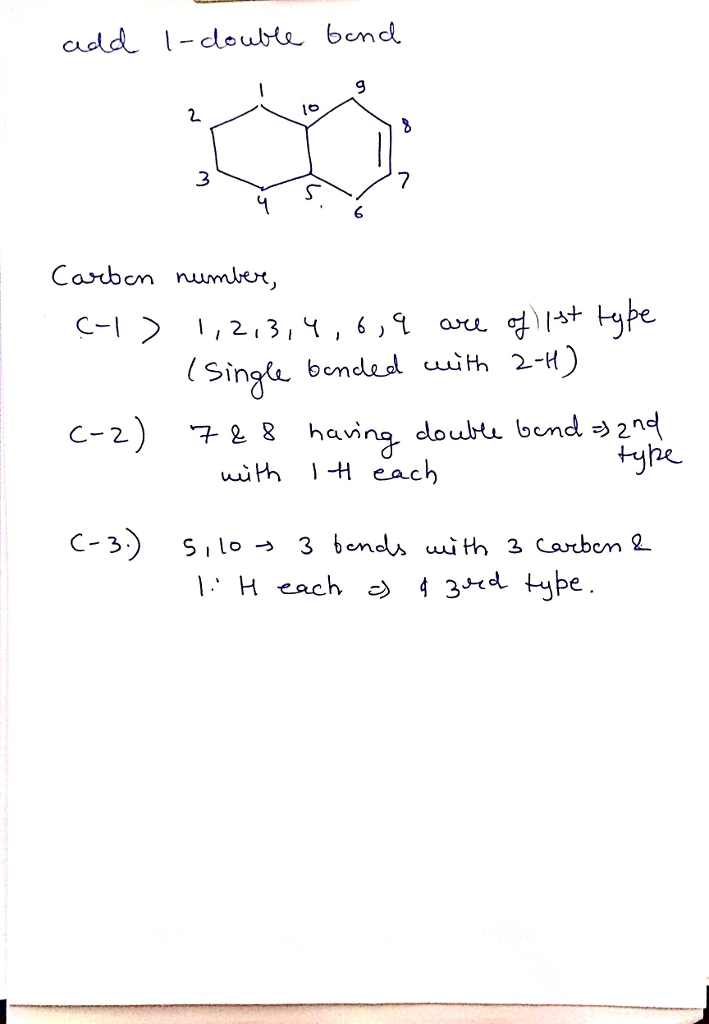#### Earn Coin

Coins can be redeemed for fabulous gifts.

Similar Homework Help Questions
• ### 3 attempts loft Check my work Be sure to answer all parts. When two six-membered rings...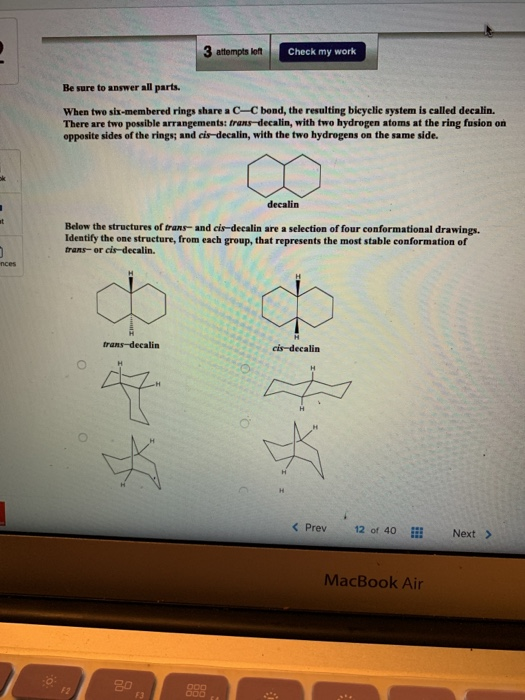3 attempts loft Check my work Be sure to answer all parts. When two six-membered rings share a C-C bond, the resulting bleyelie system is called decalin. There are two possible arrangements: frans-decalin, with two hydrogen atoms at the ring fusion on opposite sides of the rings; and cis-decalin, with the two hydrogens on the same side. decalin Below the structures of trans and cis-decalin are a selection of four conformational drawings Identify the one structure, from each group, that...

• ### unknown compound had formula C8H10, only two proton NMR signals and had 3 types of carbon...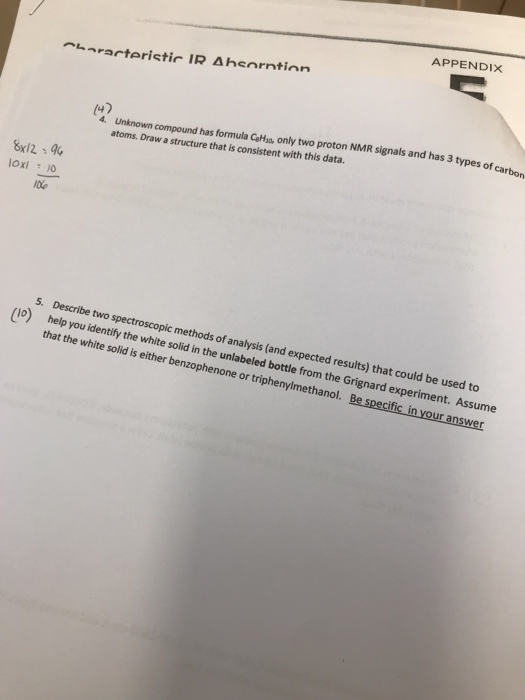unknown compound had formula C8H10, only two proton NMR signals and had 3 types of carbon atoms. draw a structure that is consistent with this data APPENDIX Unknown compound has formula CeHso, only two proton NMR signals and has 3 atoms. 4. Unknown Draw a structure that is consistent with this data. 8x124 5. Describe two spectroscopic methods of analysis (and expected results) that could be used to (Io) help you identify the white solid in the unlabeled bottle from...

• ### Mothballs are composed of naphthalene

Mothballs are composed of naphthalene, C10H8, a molecule of which consists of two six membered rings of carbon fused along an edge, as shown in this incompleteLewis structure:HHHCCHCCCCCCHCCHHH*** there should be a hexagon on either side connecting all the C's ****a) write the complete Lewis structures for naphthalene

• ### Draw two Lewis structures for a compound with the formula C, H, No atom bears a...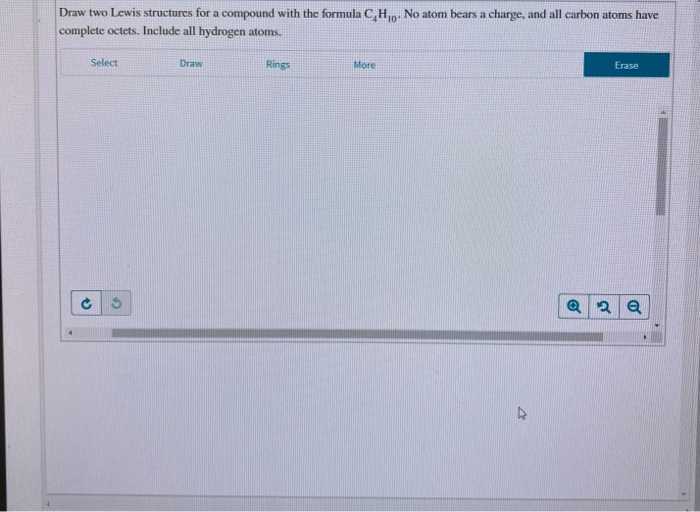Draw two Lewis structures for a compound with the formula C, H, No atom bears a charge, and all carbon atoms have complete octets. Include all hydrogen atoms. Select Draw Rings More Erase c 5 @ 2

• ### Question 31 5 pts Benzene (below) contains a six-membered ring of carbon atoms with one hydrogen...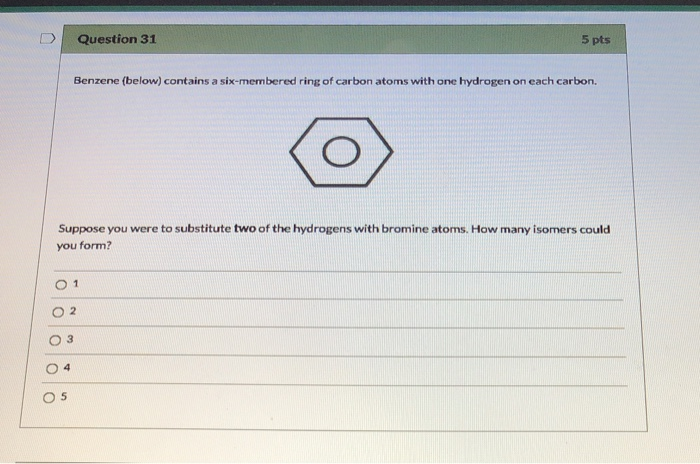Question 31 5 pts Benzene (below) contains a six-membered ring of carbon atoms with one hydrogen on each carbon. Suppose you were to substitute two of the hydrogens with bromine atoms. How many isomers could you form? 0 1 0.00

• ### A compound composed of only carbon and hydrogen is 7.743% hydrogen by mass. The compound has...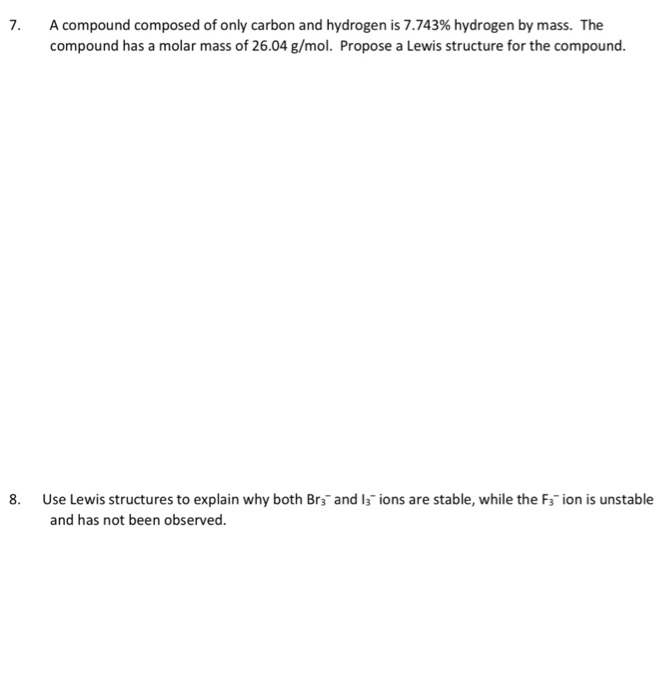A compound composed of only carbon and hydrogen is 7.743% hydrogen by mass. The compound has a molar mass of 26.04 g/mol. Propose a Lewis structure for the compound. 8. Use Lewis structures to explain why both Br_3- and l_j" ions are stable, while the F_3 ion is unstable and has not been observed.

• ### 3) A compound that is composed of only carbon and hydrogen is known as a(n): A)...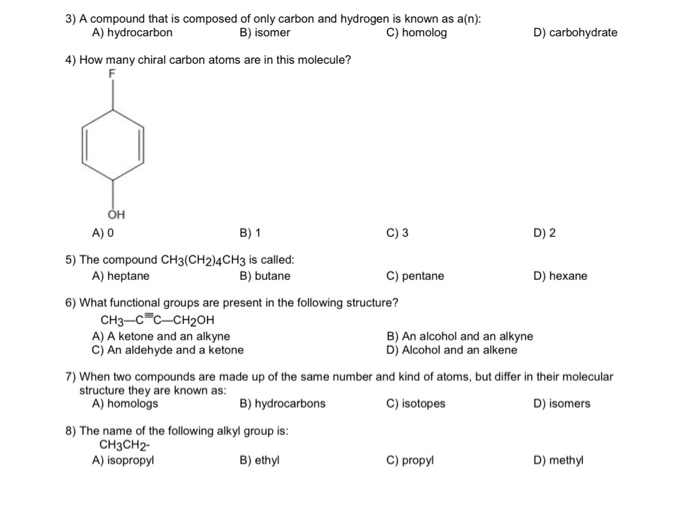3) A compound that is composed of only carbon and hydrogen is known as a(n): A) hydrocarbon B) isomer C) homolog D) carbohydrate 4) How many chiral carbon atoms are in this molecule? Он A) 0 B) 1 C) 3 D) 2 5) The compound CH3(CH2)4CH3 is called: A) heptane B) butane C) pentane D) hexane 6) What functional groups are present in the following structure? СHз-с"с-СH2ОН A) A ketone and an alkyne An aldehyde and a ketone B) An...

• ### CHAPTERS Practice Problems 6. An unknown gaseous hydrocarbon (compound composed of carbon and thydrogen only) conai...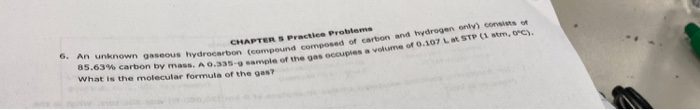CHAPTERS Practice Problems 6. An unknown gaseous hydrocarbon (compound composed of carbon and thydrogen only) conaists of 85.63% carbon by mass. A 0.335-g sample of the gas occupies a volume of 0.107 Lat STP (1 atm, oPC) What is the molecular formula of the gas7

• ### what is the empirical formula for a compound composed of 0.0892 mol of carbon, 0.0446 mol...

what is the empirical formula for a compound composed of 0.0892 mol of carbon, 0.0446 mol of hydrogen and 0.0223 mol of nitrogen?

• ### A compound composed of 3.3% H, 19.3% C, and 77.4% O has a molar mass of...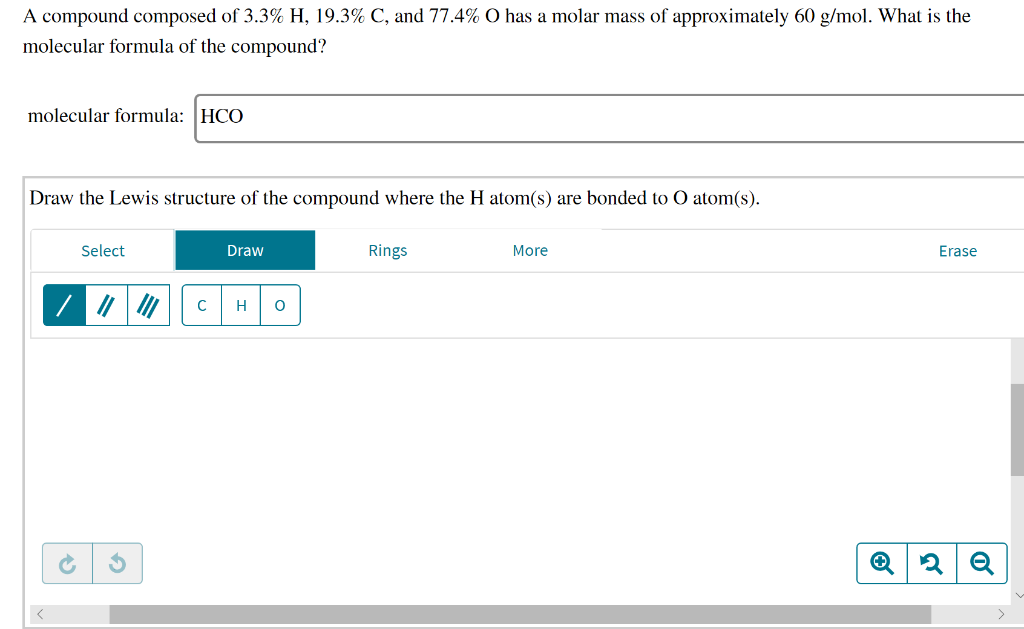A compound composed of 3.3% H, 19.3% C, and 77.4% O has a molar mass of approximately 60 g/mol. What is the molecular formula of the compound? molecular formula: HCO Draw the Lewis structure of the compound where the H atom(s) are bonded to O atom(s). Select Draw Rings More Erase How many o and a bonds are in the compound? o bonds: Activate Windows Go to Settings to activate vind a bonds:

Free Homework App